# Mean State

Period Mean (original grids) [Watt m-2]
Model Period Mean (intersection) [Watt m-2]
Model Period Mean (complement) [Watt m-2]
Benchmark Period Mean (intersection) [Watt m-2]
Benchmark Period Mean (complement) [Watt m-2]
Bias [Watt m-2]
RMSE [Watt m-2]
Phase Shift [months]
Bias Score 
RMSE Score 
Seasonal Cycle Score 
Spatial Distribution Score 
Interannual Variability Score 
Overall Score 
Benchmark [-] 453.
CLM4 [-] 456. 456. 453. 460. 3.92 12.5 1.52 0.548 0.366 0.780 1.00 0.756 0.636
CLM4.5 [-] 460. 461. 453. 460. 8.11 15.4 1.46 0.412 0.371 0.787 0.998 0.735 0.612
CLM5 [-] 462. 462. 453. 460. 9.34 15.7 1.72 0.344 0.372 0.753 0.988 0.715 0.591
Period Mean (original grids) [Watt m-2]
Model Period Mean (intersection) [Watt m-2]
Model Period Mean (complement) [Watt m-2]
Benchmark Period Mean (intersection) [Watt m-2]
Benchmark Period Mean (complement) [Watt m-2]
Bias [Watt m-2]
RMSE [Watt m-2]
Phase Shift [months]
Bias Score 
RMSE Score 
Seasonal Cycle Score 
Spatial Distribution Score 
Interannual Variability Score 
Overall Score 
Benchmark [-] 471.
CLM4 [-] 474. 475. 471. 456. 3.22 17.5 0.681 0.691 0.498 0.948 0.996 0.757 0.731
CLM4.5 [-] 480. 480. 471. 456. 8.58 20.4 0.621 0.574 0.510 0.954 0.942 0.737 0.704
CLM5 [-] 481. 482. 471. 456. 10.1 20.9 0.713 0.562 0.498 0.945 0.949 0.707 0.693
Period Mean (original grids) [Watt m-2]
Model Period Mean (intersection) [Watt m-2]
Model Period Mean (complement) [Watt m-2]
Benchmark Period Mean (intersection) [Watt m-2]
Benchmark Period Mean (complement) [Watt m-2]
Bias [Watt m-2]
RMSE [Watt m-2]
Phase Shift [months]
Bias Score 
RMSE Score 
Seasonal Cycle Score 
Spatial Distribution Score 
Interannual Variability Score 
Overall Score 
Benchmark [-] 443.
CLM4 [-] 446. 446. 443. 444. 2.74 15.3 0.853 0.659 0.514 0.896 0.986 0.653 0.703
CLM4.5 [-] 452. 452. 443. 444. 8.38 18.2 0.844 0.530 0.515 0.897 0.977 0.648 0.680
CLM5 [-] 451. 451. 443. 444. 7.63 17.9 1.14 0.566 0.494 0.853 0.988 0.608 0.667
Period Mean (original grids) [Watt m-2]
Model Period Mean (intersection) [Watt m-2]
Model Period Mean (complement) [Watt m-2]
Benchmark Period Mean (intersection) [Watt m-2]
Benchmark Period Mean (complement) [Watt m-2]
Bias [Watt m-2]
RMSE [Watt m-2]
Phase Shift [months]
Bias Score 
RMSE Score 
Seasonal Cycle Score 
Spatial Distribution Score 
Interannual Variability Score 
Overall Score 
Benchmark [-] 276.
CLM4 [-] 274. 274. 275. 292. 1.58 15.1 0.0560 0.914 0.803 0.996 0.997 0.823 0.889
CLM4.5 [-] 274. 274. 275. 292. 1.52 15.1 0.0575 0.912 0.803 0.996 0.993 0.805 0.885
CLM5 [-] 275. 275. 275. 292. 3.64 16.0 0.0557 0.901 0.799 0.996 0.989 0.793 0.880
Period Mean (original grids) [Watt m-2]
Model Period Mean (intersection) [Watt m-2]
Model Period Mean (complement) [Watt m-2]
Benchmark Period Mean (intersection) [Watt m-2]
Benchmark Period Mean (complement) [Watt m-2]
Bias [Watt m-2]
RMSE [Watt m-2]
Phase Shift [months]
Bias Score 
RMSE Score 
Seasonal Cycle Score 
Spatial Distribution Score 
Interannual Variability Score 
Overall Score 
Benchmark [-] 456.
CLM4 [-] 451. 451. 453. 465. -0.292 8.68 1.84 0.385 0.386 0.717 0.918 0.713 0.584
CLM4.5 [-] 454. 454. 453. 465. 1.47 9.23 1.82 0.354 0.395 0.721 0.925 0.707 0.583
CLM5 [-] 458. 458. 453. 465. 5.84 10.1 1.76 0.314 0.411 0.732 0.934 0.717 0.587
Period Mean (original grids) [Watt m-2]
Model Period Mean (intersection) [Watt m-2]
Model Period Mean (complement) [Watt m-2]
Benchmark Period Mean (intersection) [Watt m-2]
Benchmark Period Mean (complement) [Watt m-2]
Bias [Watt m-2]
RMSE [Watt m-2]
Phase Shift [months]
Bias Score 
RMSE Score 
Seasonal Cycle Score 
Spatial Distribution Score 
Interannual Variability Score 
Overall Score 
Benchmark [-] 306.
CLM4 [-] 312. 312. 306. 329. 6.72 13.0 0.0202 0.898 0.861 0.999 0.998 0.891 0.918
CLM4.5 [-] 313. 312. 306. 329. 7.02 13.1 0.0199 0.896 0.860 0.999 1.00 0.896 0.919
CLM5 [-] 316. 315. 306. 329. 10.1 14.8 0.0215 0.860 0.862 0.999 0.997 0.891 0.912
Period Mean (original grids) [Watt m-2]
Model Period Mean (intersection) [Watt m-2]
Model Period Mean (complement) [Watt m-2]
Benchmark Period Mean (intersection) [Watt m-2]
Benchmark Period Mean (complement) [Watt m-2]
Bias [Watt m-2]
RMSE [Watt m-2]
Phase Shift [months]
Bias Score 
RMSE Score 
Seasonal Cycle Score 
Spatial Distribution Score 
Interannual Variability Score 
Overall Score 
Benchmark [-] 362.
CLM4 [-] 360. 360. 361. 395. 1.19 14.3 0.102 0.879 0.821 0.993 0.985 0.745 0.874
CLM4.5 [-] 361. 360. 361. 395. 1.65 14.2 0.0991 0.880 0.823 0.993 0.983 0.741 0.874
CLM5 [-] 364. 363. 361. 395. 4.75 15.4 0.151 0.860 0.817 0.990 0.991 0.730 0.867
Period Mean (original grids) [Watt m-2]
Model Period Mean (intersection) [Watt m-2]
Model Period Mean (complement) [Watt m-2]
Benchmark Period Mean (intersection) [Watt m-2]
Benchmark Period Mean (complement) [Watt m-2]
Bias [Watt m-2]
RMSE [Watt m-2]
Phase Shift [months]
Bias Score 
RMSE Score 
Seasonal Cycle Score 
Spatial Distribution Score 
Interannual Variability Score 
Overall Score 
Benchmark [-] 450.
CLM4 [-] 445. 446. 450. 463. -1.23 16.8 0.656 0.690 0.553 0.931 0.999 0.667 0.732
CLM4.5 [-] 450. 451. 450. 463. 2.82 17.5 0.654 0.658 0.560 0.932 1.00 0.658 0.728
CLM5 [-] 452. 452. 450. 463. 4.66 17.7 0.767 0.653 0.557 0.922 1.00 0.627 0.719
Period Mean (original grids) [Watt m-2]
Model Period Mean (intersection) [Watt m-2]
Model Period Mean (complement) [Watt m-2]
Benchmark Period Mean (intersection) [Watt m-2]
Benchmark Period Mean (complement) [Watt m-2]
Bias [Watt m-2]
RMSE [Watt m-2]
Phase Shift [months]
Bias Score 
RMSE Score 
Seasonal Cycle Score 
Spatial Distribution Score 
Interannual Variability Score 
Overall Score 
Benchmark [-] 275.
CLM4 [-] 280. 280. 275. 280. 6.13 14.0 0.0290 0.895 0.824 0.998 0.996 0.874 0.902
CLM4.5 [-] 279. 280. 275. 280. 5.62 13.6 0.0329 0.902 0.825 0.997 0.997 0.855 0.900
CLM5 [-] 282. 282. 275. 280. 7.91 15.3 0.0355 0.875 0.817 0.997 0.996 0.851 0.892
Period Mean (original grids) [Watt m-2]
Model Period Mean (intersection) [Watt m-2]
Model Period Mean (complement) [Watt m-2]
Benchmark Period Mean (intersection) [Watt m-2]
Benchmark Period Mean (complement) [Watt m-2]
Bias [Watt m-2]
RMSE [Watt m-2]
Phase Shift [months]
Bias Score 
RMSE Score 
Seasonal Cycle Score 
Spatial Distribution Score 
Interannual Variability Score 
Overall Score 
Benchmark [-] 448.
CLM4 [-] 447. 447. 450. 423. -5.23 14.5 0.334 0.770 0.719 0.974 0.996 0.728 0.818
CLM4.5 [-] 448. 448. 450. 423. -4.68 14.6 0.329 0.765 0.718 0.974 0.996 0.720 0.815
CLM5 [-] 449. 450. 450. 423. -3.08 14.6 0.337 0.785 0.707 0.974 0.994 0.702 0.812
Period Mean (original grids) [Watt m-2]
Model Period Mean (intersection) [Watt m-2]
Model Period Mean (complement) [Watt m-2]
Benchmark Period Mean (intersection) [Watt m-2]
Benchmark Period Mean (complement) [Watt m-2]
Bias [Watt m-2]
RMSE [Watt m-2]
Phase Shift [months]
Bias Score 
RMSE Score 
Seasonal Cycle Score 
Spatial Distribution Score 
Interannual Variability Score 
Overall Score 
Benchmark [-] 364.
CLM4 [-] 360. 360. 362. 385. 1.09 10.8 0.244 0.871 0.799 0.984 0.995 0.838 0.881
CLM4.5 [-] 359. 359. 362. 385. 0.572 10.1 0.218 0.881 0.807 0.985 0.994 0.842 0.886
CLM5 [-] 363. 363. 362. 385. 3.66 10.8 0.476 0.859 0.809 0.968 0.993 0.830 0.878
Period Mean (original grids) [Watt m-2]
Model Period Mean (intersection) [Watt m-2]
Model Period Mean (complement) [Watt m-2]
Benchmark Period Mean (intersection) [Watt m-2]
Benchmark Period Mean (complement) [Watt m-2]
Bias [Watt m-2]
RMSE [Watt m-2]
Phase Shift [months]
Bias Score 
RMSE Score 
Seasonal Cycle Score 
Spatial Distribution Score 
Interannual Variability Score 
Overall Score 
Benchmark [-] 397.
CLM4 [-] 375. 375. 376. 406. 0.774 14.9 0.471 0.748 0.685 0.947 0.999 0.759 0.804
CLM4.5 [-] 377. 377. 376. 406. 2.18 15.4 0.462 0.721 0.687 0.948 0.999 0.753 0.799
CLM5 [-] 380. 380. 376. 406. 4.60 16.3 0.517 0.699 0.685 0.943 0.998 0.739 0.792
Period Mean (original grids) [Watt m-2]
Model Period Mean (intersection) [Watt m-2]
Model Period Mean (complement) [Watt m-2]
Benchmark Period Mean (intersection) [Watt m-2]
Benchmark Period Mean (complement) [Watt m-2]
Bias [Watt m-2]
RMSE [Watt m-2]
Phase Shift [months]
Bias Score 
RMSE Score 
Seasonal Cycle Score 
Spatial Distribution Score 
Interannual Variability Score 
Overall Score 
Benchmark [-] 464.
CLM4 [-] 448. 448. 465. 441. -17.1 24.8 0.424 0.692 0.736 0.967 0.977 0.660 0.795
CLM4.5 [-] 448. 448. 465. 441. -16.7 24.5 0.415 0.695 0.737 0.968 0.976 0.656 0.795
CLM5 [-] 450. 449. 465. 441. -15.4 24.1 0.443 0.709 0.731 0.965 0.973 0.645 0.792
Period Mean (original grids) [Watt m-2]
Model Period Mean (intersection) [Watt m-2]
Model Period Mean (complement) [Watt m-2]
Benchmark Period Mean (intersection) [Watt m-2]
Benchmark Period Mean (complement) [Watt m-2]
Bias [Watt m-2]
RMSE [Watt m-2]
Phase Shift [months]
Bias Score 
RMSE Score 
Seasonal Cycle Score 
Spatial Distribution Score 
Interannual Variability Score 
Overall Score 
Benchmark [-] 278.
CLM4 [-] 283. 283. 277. 289. 5.82 12.9 0.0629 0.885 0.835 0.996 0.994 0.835 0.897
CLM4.5 [-] 283. 282. 277. 289. 5.52 12.8 0.0535 0.889 0.835 0.996 0.996 0.823 0.896
CLM5 [-] 285. 285. 277. 289. 8.39 14.5 0.0541 0.857 0.830 0.996 0.995 0.817 0.887
Period Mean (original grids) [Watt m-2]
Model Period Mean (intersection) [Watt m-2]
Model Period Mean (complement) [Watt m-2]
Benchmark Period Mean (intersection) [Watt m-2]
Benchmark Period Mean (complement) [Watt m-2]
Bias [Watt m-2]
RMSE [Watt m-2]
Phase Shift [months]
Bias Score 
RMSE Score 
Seasonal Cycle Score 
Spatial Distribution Score 
Interannual Variability Score 
Overall Score 
Benchmark [-] 444.
CLM4 [-] 445. 445. 442. 455. 7.04 14.7 0.596 0.552 0.578 0.937 0.944 0.743 0.722
CLM4.5 [-] 445. 445. 442. 455. 6.38 14.1 0.610 0.581 0.576 0.934 0.978 0.734 0.730
CLM5 [-] 446. 446. 442. 455. 7.88 14.4 0.674 0.552 0.584 0.934 0.989 0.719 0.727
Period Mean (original grids) [Watt m-2]
Model Period Mean (intersection) [Watt m-2]
Model Period Mean (complement) [Watt m-2]
Benchmark Period Mean (intersection) [Watt m-2]
Benchmark Period Mean (complement) [Watt m-2]
Bias [Watt m-2]
RMSE [Watt m-2]
Phase Shift [months]
Bias Score 
RMSE Score 
Seasonal Cycle Score 
Spatial Distribution Score 
Interannual Variability Score 
Overall Score 
Benchmark [-] 386.
CLM4 [-] 384. 384. 384. 429. 2.59 8.87 0.141 0.910 0.852 0.989 0.995 0.880 0.913
CLM4.5 [-] 386. 386. 384. 429. 4.32 9.66 0.149 0.885 0.855 0.989 0.998 0.877 0.910
CLM5 [-] 390. 390. 384. 429. 8.58 11.9 0.176 0.825 0.859 0.988 1.00 0.865 0.899
Period Mean (original grids) [Watt m-2]
Model Period Mean (intersection) [Watt m-2]
Model Period Mean (complement) [Watt m-2]
Benchmark Period Mean (intersection) [Watt m-2]
Benchmark Period Mean (complement) [Watt m-2]
Bias [Watt m-2]
RMSE [Watt m-2]
Phase Shift [months]
Bias Score 
RMSE Score 
Seasonal Cycle Score 
Spatial Distribution Score 
Interannual Variability Score 
Overall Score 
Benchmark [-] 319.
CLM4 [-] 324. 324. 318. 343. 6.95 12.4 0.0825 0.875 0.842 0.994 0.992 0.852 0.899
CLM4.5 [-] 324. 324. 318. 343. 6.93 12.6 0.0830 0.873 0.838 0.994 0.995 0.842 0.897
CLM5 [-] 327. 327. 318. 343. 10.1 14.6 0.138 0.832 0.838 0.991 0.990 0.839 0.888
Period Mean (original grids) [Watt m-2]
Model Period Mean (intersection) [Watt m-2]
Model Period Mean (complement) [Watt m-2]
Benchmark Period Mean (intersection) [Watt m-2]
Benchmark Period Mean (complement) [Watt m-2]
Bias [Watt m-2]
RMSE [Watt m-2]
Phase Shift [months]
Bias Score 
RMSE Score 
Seasonal Cycle Score 
Spatial Distribution Score 
Interannual Variability Score 
Overall Score 
Benchmark [-] 376.
CLM4 [-] 377. 378. 376. 379. 2.01 15.1 0.0825 0.873 0.802 0.994 0.984 0.741 0.866
CLM4.5 [-] 377. 378. 376. 379. 2.27 14.5 0.0817 0.874 0.813 0.995 0.983 0.748 0.871
CLM5 [-] 380. 381. 376. 379. 5.26 16.3 0.108 0.854 0.798 0.993 0.979 0.711 0.856
Period Mean (original grids) [Watt m-2]
Model Period Mean (intersection) [Watt m-2]
Model Period Mean (complement) [Watt m-2]
Benchmark Period Mean (intersection) [Watt m-2]
Benchmark Period Mean (complement) [Watt m-2]
Bias [Watt m-2]
RMSE [Watt m-2]
Phase Shift [months]
Bias Score 
RMSE Score 
Seasonal Cycle Score 
Spatial Distribution Score 
Interannual Variability Score 
Overall Score 
Benchmark [-] 449.
CLM4 [-] 452. 452. 449. 458. 3.54 11.8 1.29 0.579 0.391 0.812 0.900 0.660 0.622
CLM4.5 [-] 455. 455. 449. 458. 6.23 12.9 1.19 0.490 0.393 0.832 0.891 0.653 0.609
CLM5 [-] 457. 457. 449. 458. 7.86 13.4 1.25 0.424 0.399 0.822 0.935 0.629 0.601
Period Mean (original grids) [Watt m-2]
Model Period Mean (intersection) [Watt m-2]
Model Period Mean (complement) [Watt m-2]
Benchmark Period Mean (intersection) [Watt m-2]
Benchmark Period Mean (complement) [Watt m-2]
Bias [Watt m-2]
RMSE [Watt m-2]
Phase Shift [months]
Bias Score 
RMSE Score 
Seasonal Cycle Score 
Spatial Distribution Score 
Interannual Variability Score 
Overall Score 
Benchmark [-] 440.
CLM4 [-] 443. 443. 440. 430. 2.20 12.5 0.736 0.697 0.592 0.900 0.966 0.770 0.753
CLM4.5 [-] 446. 446. 440. 430. 4.76 13.0 0.867 0.657 0.592 0.884 0.958 0.781 0.744
CLM5 [-] 448. 448. 440. 430. 6.99 12.9 0.794 0.659 0.605 0.895 0.980 0.735 0.746
Period Mean (original grids) [Watt m-2]
Model Period Mean (intersection) [Watt m-2]
Model Period Mean (complement) [Watt m-2]
Benchmark Period Mean (intersection) [Watt m-2]
Benchmark Period Mean (complement) [Watt m-2]
Bias [Watt m-2]
RMSE [Watt m-2]
Phase Shift [months]
Bias Score 
RMSE Score 
Seasonal Cycle Score 
Spatial Distribution Score 
Interannual Variability Score 
Overall Score 
Benchmark [-] 387.
CLM4 [-] 384. 383. 386. 394. -2.07 16.5 0.563 0.647 0.626 0.942 0.998 0.735 0.762
CLM4.5 [-] 386. 385. 386. 394. -0.400 16.6 0.560 0.640 0.630 0.942 0.999 0.730 0.762
CLM5 [-] 387. 386. 386. 394. 0.770 16.4 0.562 0.638 0.632 0.940 0.997 0.707 0.758

# Temporally integrated period mean

BENCHMARK MEAN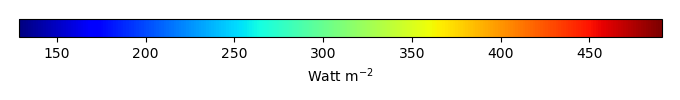MODEL MEANBIAS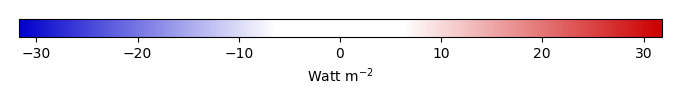BIAS SCORERMSE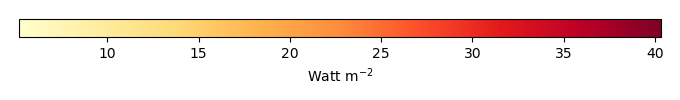RMSE SCOREBENCHMARK INTERANNUAL VARIABILITY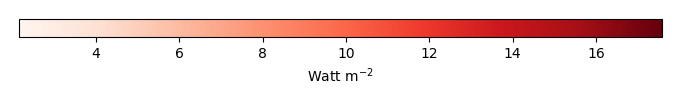MODEL INTERANNUAL VARIABILITYINTERANNUAL VARIABILITY SCOREBENCHMARK MAX MONTHMODEL MAX MONTHDIFFERENCE IN MAX MONTH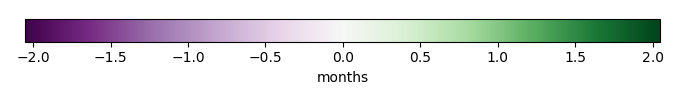SEASONAL CYCLE SCORESPATIAL TAYLOR DIAGRAMMODEL COLORS# Spatially integrated regional mean

MODEL COLORSREGIONAL MEANANNUAL CYCLEMONTHLY ANOMALYANNUAL CYCLE# All Models

BenchmarkCLM4CLM4.5CLM5# Data Information

creation_date: Mon Jun 30 23:35:20 PDT 2014

source_file: This product is generated from monthly 1 degree GEWEX SRB Radiation observations